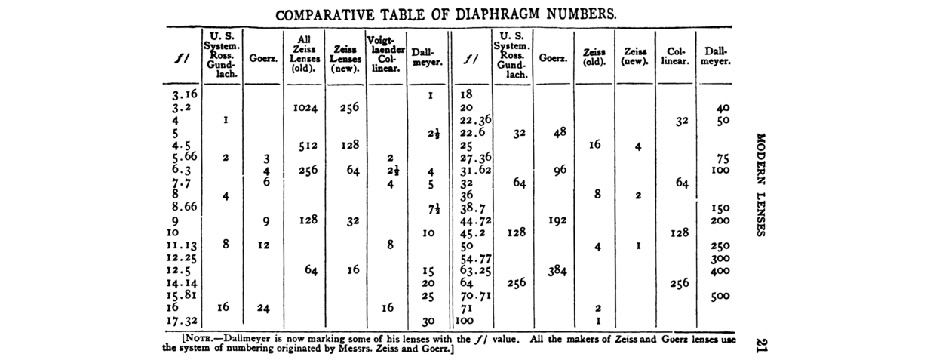Top
The Difference Between F Stops and T Stops – The Camera Forum®
49372

# The Difference Between F Stops and T Stops## The Difference Between F Stops and T Stops

Matt Granger, from ThatNikonGuy.Com digs into the difference between F Stops and T Stops in this short presentation.  Just what is that difference, and is that difference important to us, you mite ask?Diagram of decreasing apertures, that is, increasing f-numbers, in one-stop increments; each aperture has half the light gathering area of the previous one. Source:Wikipedia Commons

In the science of optics, the f-number (sometimes called focal ratiof-ratiof-stop, or relative aperture) of an optical system is the ratio of the lens’s focal length to the diameter of the entrance pupil at the front of the lens.  It is a dimensionless number that is used by most photographers and others involved in video production, a quantitative measure of lens speed, and an important concept in photography overall. The actual mathematical formula this photographer shorthand derives from is:

Solve for f-number N is given by$N = \frac{f}{D} \$

where$f$ is the focal length, and$D$ is the diameter of the entrance pupil (effective aperture). It is customary to write f-numbers preceded by f/, which forms a mathematical expression of the entrance pupil diameter at the front of the lens, in terms of f and N. For example, if a lens’s focal length is 20 mm and its entrance pupil diameter is 10 mm, the f-number is 2 and the aperture diameter is f/2.

But hang on, there is also a T Stop that can be calculated.  A T-stop (the “T” is a shorthand for Transmission stops) is an f-number adjusted to account for light transmission efficiency (transmittance). A lens with a T-stop of N projects an image of the same brightness as an ideal lens with 100% transmittance and an f-number of N. As in our example above, an f/2.0 lens with transmittance of 75% has a T-stop of 2.3. Since real lenses always have transmittances of less than 100%, a lens’s T-stop is always greater than its f-number, though the difference can be so slight that it is often not reported as a difference.

Lens transmittances of 60%–90% are much more typical, so T-stops are sometimes used instead of f-numbers to more accurately determine exposure, as with external light meters. T-stops are often used in the fields of film cinematography and video production, where many images are seen in rapid succession and even small changes in exposure will be immediately noticeable as a difference by the viewing audience. This is why Cinema camera lenses are typically calibrated in T-stops instead of F-numbers. In still photography, the need for rigorous and absolute consistency of all lenses and cameras is not as important, as slight differences in exposure are less critical than in moving imagery.